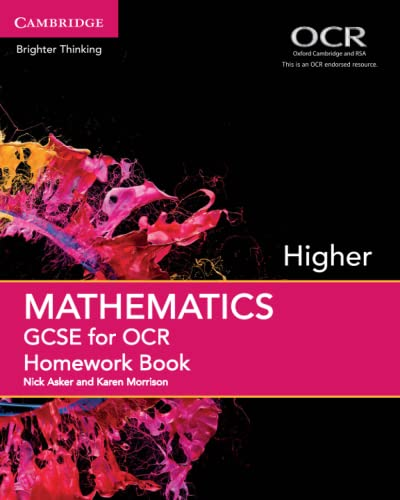Free Shipping on all orders in Australia
Over 7 million books in stock
We aim to be carbon neutral by 2022# GCSE Mathematics for OCR Higher Homework Book by Nick Asker

Condition - Very Good
\$16,49
Only 1 left

## Summary

A new series of bespoke, full-coverage resources developed for the 2015 GCSE Mathematics qualifications.

## GCSE Mathematics for OCR Higher Homework Book Summary

### GCSE Mathematics for OCR Higher Homework Book by Nick Asker

A new series of bespoke, full-coverage resources developed for the 2015 GCSE Mathematics qualifications. Endorsed for the OCR J560 GCSE Mathematics Higher tier specification for first teaching from 2015, our Homework Book is an ideal companion to the OCR Higher tier Student Book and can be used as a standalone resource. With exercises that correspond to each section of the Student Book, it offers a wealth of additional questions for practice and consolidation. Our Homework Books contain a breadth and depth of questions covering a variety of skills, including problem-solving and mathematical reasoning, as well as extensive drill questions. Answers to all questions are available free on the Cambridge University Press UK Schools website.

## Table of Contents

Introduction; 1. Basic calculation skills; 2. Whole number theory; 3. Algebraic expressions; 4. Functions and sequences; 5. Properties of shapes and solids; 6. Construction and loci; 7. Further algebraic expressions; 8. Equations; 9. Angles; 10. Fractions; 11. Decimals; 12. Units and measurement; 13. Percentages; 14. Algebraic formulae; 15. Perimeter; 16. Area; 17. Approximation and estimation; 18. Straight-line graphs; 19. Graphs of equations and functions; 20. Three-dimensional shapes; 21. Volume and surface area; 22. Calculations with ratio; 23. Basic probability and experiments; 24. Combined events and probability diagrams; 25. Powers and roots; 26. Standard form; 27. Surds; 28. Plane vector geometry; 29. Plane isometric transformations; 30. Congruent triangles; 31. Similarity; 32. Pythagoras' theorem; 33. Trigonometry; 34. Circle theorems; 35. Discrete growth and decay; 36. Direct and inverse proportion; 37. Collecting and displaying data; 38. Analysing data; 39. Interpreting graphs; 40. Algebraic inequalities; 41. Transformations of curves and their equations; Glossary; Index.

## Additional information

GOR007354681
GCSE Mathematics for OCR Higher Homework Book by Nick Asker
Used - Very Good
Paperback
Cambridge University Press
2015-06-18
152
1107496926
9781107496927
N/A
Book picture is for illustrative purposes only, actual binding, cover or edition may vary.
This is a used book - there is no escaping the fact it has been read by someone else and it will show signs of wear and previous use. Overall we expect it to be in very good condition, but if you are not entirely satisfied please get in touch with us